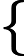Study of mathematics online.
Study math with us and make sure that "Mathematics is easy!"

# Linearly dependent and linearly independent vectors

Definition. A linear combination of vectors a1, ..., an with coefficients x1, ..., xn is a vector

x1a1 + ... + xnan.

Definition. A linear combination x1a1 + ... + xnan are called trivial if all the coefficients x1, ..., xn are zero.
Definition. A linear combination x1a1 + ... + xnan are called nontrivial if at least one of the coefficients x1, ..., xn is not zero.
Definition. The vectors a1, ..., an are called linearly independent if there are no non-trivial combination of these vectors equal to the zero vector.

That is, the vector a1, ..., an are linearly independent if x1a1 + ... + xnan = 0 if and only if x1 = 0, ..., xn = 0.

Definition. The vectors a1, ..., an are called linearly dependent if there exists a non-trivial combination of these vectors is equal to the zero vector.

## Linearly dependent vectors properties:

• For 2-D and 3-D vectors.
Two linearly dependent vectors are collinear. (Collinear vectors are linearly dependent.)
• For 3-D vectors.
Three linear dependence vectors are coplanar. (Three coplanar vectors are linearly dependent.)
• For an n-dimensional vectors.
n + 1 vectors always linearly dependent.

## Linearly dependent and linearly independent vectors examples:

Example 1. Check whether the vectors a = {3; 4; 5}, b = {-3; 0; 5}, c = {4; 4; 4}, d = {3; 4; 0} are linearly independent.

Solution:

The vectors are linearly dependent, since the dimension of the vectors smaller than the number of vectors.

Example 2. Check whether the vectors a = {1; 1; 1}, b = {1; 2; 0}, c = {0; -1; 1} are linearly independent.

Solution: Calculate the coefficients in which a linear combination of these vectors is equal to the zero vector.

x1a + x2b + x3c1 = 0

This vector equation can be written as a system of linear equationsx1 + x2 = 0 x1 + 2x2 - x3 = 0 x1 + x3 = 0

Solve this system using the Gauss method1 1 0 0~ 1 2 -1 0 1 0 1 0

from 2 row we subtract the 1-th row;from 3 row we subtract the 1-th row:

 ~1 1 0 0~1 1 0 0~ 1 - 1 2 - 1 -1 - 0 0 - 0 0 1 -1 0 1 - 1 0 - 1 1 - 0 0 - 0 0 -1 1 0

from 1 row we subtract the 2 row; for 3 row add 2 row:

 ~1 - 0 1 - 1 0 - (-1) 0 - 0~1 0 1 00 1 -1 0 0 1 -1 0 0 + 0 -1 + 1 1 + (-1) 0 + 0 0 0 0 0

This solution shows that the system has many solutions, ie exist nonzero combination of numbers x1, x2, x3 such that the linear combination of a, b, c is equal to the zero vector, for example:

-a + b + c = 0

means vectors a, b, c are linearly dependent.

Answer: vectors a, b, c are linearly dependent.

Example 3. Check whether the vectors a = {1; 1; 1}, b = {1; 2; 0}, c = {0; -1; 2} are linearly independent.

Solution: Calculate the coefficients in which a linear combination of these vectors is equal to the zero vector.

x1a + x2b + x3c1 = 0

This vector equation can be written as a system of linear equationsx1 + x2 = 0 x1 + 2x2 - x3 = 0 x1 + 2x3 = 0

Solve this system using the Gauss method1 1 0 0~ 1 2 -1 0 1 0 2 0

from 2 row we subtract the 1-th row;from 3 row we subtract the 1-th row:

 ~1 1 0 0~1 1 0 0~ 1 - 1 2 - 1 -1 - 0 0 - 0 0 1 -1 0 1 - 1 0 - 1 2 - 0 0 - 0 0 -1 2 0

from 1 row we subtract the 2 row; for 3 row add 2 row:

 ~1 - 0 1 - 1 0 - (-1) 0 - 0~1 0 1 0~ 0 1 -1 0 0 1 -1 0 0 + 0 -1 + 1 2 + (-1) 0 + 0 0 0 1 0

from 1 row we subtract the 3 row; for 2 row add 3 row:

 ~1 - 0 0 - 0 1 - 1 0 - 0~1 0 0 00 + 0 1 + 0 -1 + 1 0 + 0 0 1 0 0 0 0 1 0 0 0 1 0

This means that the system has a unique solution x1 = 0, x2 = 0, x3 = 0, and the vectors a, b, c are linearly independent.

Answer: vectors a, b, c are linearly independent.

Add the comment

 0